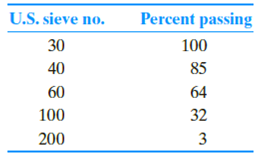Chapter 7, Problem 7.17PPrinciples of Geotechnical Enginee...

9th Edition
Braja M. Das + 1 other
ISBN: 9781305970939

Solutions

Chapter
SectionPrinciples of Geotechnical Enginee...

9th Edition
Braja M. Das + 1 other
ISBN: 9781305970939
Textbook Problem

The grain-size analysis data for a sand is given in the following table. Estimate the hydraulic conductivity of the sand at a void ratio of 0.77. Use Eq. (7.30) and SF = 6.5.To determine

Find the hydraulic conductivity of the sand at a void ratio of 0.77.

Explanation

Given information:

The given void ratio (e) is 0.77.

The shape factor SF is 6.5.

Calculation:

Determine the fraction between two consecutive sieves for first two sieve numbers using the relation.

Fraction=(%passing)30(%passing)40

Here, (%passing)30 is the percent passing for sieve number 30 and (%passing)40 is the percent passing for sieve number 40.

Substitute 100 for (%passing)30 and 85 for (%passing)40.

(fi)30,40=10085=15

Similarly, calculate the fraction between two consecutive sieves for remaining consecutive sieves.

Summarize the calculated values as in Table (1).

 Sieve No. Opening (cm) Percent passing Fraction between two 30 0.06 100 15 40 0.0425 85 21 60 0.02 64 32 100 0.015 32 29 200 0.0075 3

Determine the fraction between sieve Nos. 30 and 40 using the relation.

Sieve30and40=(fi)30,40Dli0.404×Dsi0.595

Here, Dli is the opening size for larger sieve and Dsi is the opening size for smaller sieve.

Substitute 15 for (fi)30,40, 0.06 cm for Dli, and 0.0425 cm for Dsi.

Sieve30and40=150.060.404×0.04250.595=306.07

Determine the fraction between sieve Nos. 40 and 60 using the relation.

Sieve40and60=(fi)40,60Dli0.404×Dsi0.595

Substitute 21 for (fi)30,40, 0.0425 cm for Dli, and 0.02 cm for Dsi.

Sieve40and60=210.04250.404×0.020.595=771

Still sussing out bartleby?

Check out a sample textbook solution.

See a sample solution

The Solution to Your Study Problems

Bartleby provides explanations to thousands of textbook problems written by our experts, many with advanced degrees!

Get Started

What is the correct way to check a coolant temperature sensor?

Automotive Technology: A Systems Approach (MindTap Course List)

Determine the magnitude of the couple shown.

International Edition---engineering Mechanics: Statics, 4th Edition

How can you prevent RSIs and CTS?

Enhanced Discovering Computers 2017 (Shelly Cashman Series) (MindTap Course List)

Who must be provided with safety data sheets (SDSs)?

Welding: Principles and Applications (MindTap Course List)